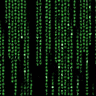# UtilityData.mac 1.3

So a lot of times I find myself wondering if something I'm trying to do is a thing. But we all know the Wiki on macroquest2.com/ is outdated more often then not these days.

Well luckily for us there was one thing there that gives us access to some information that may be lacking in details. The \${Type[datatype].Member[#]}. So we could just type \${Type[spawn].Member} and then \${Type[spawn].Member} etc 149 times in a row. But what MacroQuest user would do that silliness. I wrote a very short utility to output to the MQ2ChatWnd the information by typing /mac data dataType. IE: /mac data spawn. I went a step farther and also made it output that information to an INI file.

The layout for the INI is Data.ini, DataType, Member#=Value. Like below.

Rich (BB code):
``````[spawn]
Member1=ID
Member2=Name
Member3=Level
Member4=X
Member5=Y
Member6=Z
Member7=DistanceX
Member8=DistanceY
Member9=DistanceZ
Member10=Distance
Member11=Distance3D
Member12=DistancePredict
Member13=Next
Member14=Prev
Member16=Speed
Member17=Levitating
Member18=Sneaking
Member20=Light
Member21=Body
Member22=State
Member23=CurrentHPs
Member24=MaxHPs
Member25=PctHPs
Member26=Deity
Member28=Type
Member29=CleanName
Member30=Surname
Member31=Guild
Member32=GuildStatus
Member33=Master
Member34=Pet
Member35=Race
Member36=Class
Member38=Gender
Member39=GM
Member40=Height
Member41=MaxRange
Member42=AARank
Member43=Casting
Member44=Mount
Member45=FeetWet
Member46=Underwater
Member48=Animation
Member49=Holding
Member50=Look
Member51=N
Member52=W
Member53=U
Member54=S
Member55=E
Member56=D
Member57=DistanceN
Member58=DistanceW
Member59=DistanceU
Member60=Invis
Member62=LFG
Member64=AFK
Member65=ConColor
Member67=Standing
Member68=Sitting
Member69=Ducking
Member70=Feigning
Member71=Binding
Member72=Invited
Member73=NearestSpawn
Member74=MaxRangeTo
Member75=DisplayName
Member76=AATitle
Member78=Mark
Member79=Assist
Member80=Anonymous
Member81=Roleplaying
Member82=LineOfSight
Member84=Title
Member85=Suffix
Member86=Fleeing
Member87=Named
Member89=Moving
Member90=StandState
Member92=Stunned
Member93=Hovering
Member94=CurrentMana
Member95=MaxMana
Member96=CurrentEndurance
Member97=PctEndurance
Member98=MaxEndurance
Member99=Loc
Member100=LocYX
Member101=LocYXZ
Member102=Owner
Member103=Following
Member105=Inviter
Member106=MercID
Member107=ContractorID
Member108=PctMana
Member109=Primary
Member110=Secondary
Member111=Equipment
Member112=Targetable
Member113=PlayerState
Member114=Stuck
Member115=Aggressive
Member116=CanSplashLand
Member117=IsBerserk
Member118=pTouchingSwitch
Member119=bShowHelm
Member120=CorpseDragCount
Member121=bBetaBuffed
Member122=CombatSkillTicks
Member123=FD
Member124=InPvPArea
Member125=bAlwaysShowAura
Member126=GMRank
Member127=WarCry
Member128=IsPassenger
Member129=LastCastTime
Member130=DragNames
Member131=DraggingPlayer
Member132=bStationary
Member133=BearingToTarget
Member134=bTempPet
Member135=HoldingAnimation
Member136=Blind
Member137=LastCastNum
Member138=CollisionCounter
Member139=CeilingHeightAtCurrLocation
Member140=AssistName
Member141=SeeInvis
Member142=SpawnStatus
Member143=bWaitingForPort
Member144=EQLoc
Member145=MQLoc
Member147=FloorZ
Member148=IsSummoned
Member149=TargetOfTarget``````

The above is the result of typing /mac data spawn

I've set the macro up so that you can check any data type this way and it will produce up to 300 results if there are that many members to a datatype.

It only writes to the file if that Member# has no length. IE: if that member# doesn't have a value. IE: If I run it and there was a new member added to the datatype spawn and it now has 150 instead of 149 it would update the one spot instead of writing all 150 values.

While this is targeted at Developers, the common user can use this utility to expand their knowledge of the language.

/mac data character

would display all the things you can add to the end of \${Me}. The result would be 261 different members that can be attached to the end of \${Me}. Such as Member207=PiecesofEight. While I have no idea what PiecesofEight is, it's a member of the character data type.

A list of datatypes can be found https://macroquest2.com/wiki/index.php/Data_Types

Hope this helps someone.

~ChatWithThisName

--Update--

I've gone through the trouble of going through each data type as it was found in MQ2DataTypes.h in the test source to generate a comprehensive file. It's attached as Data.ini
Author
ChatWithThisName
First release
Last update
Rating
0 ratings